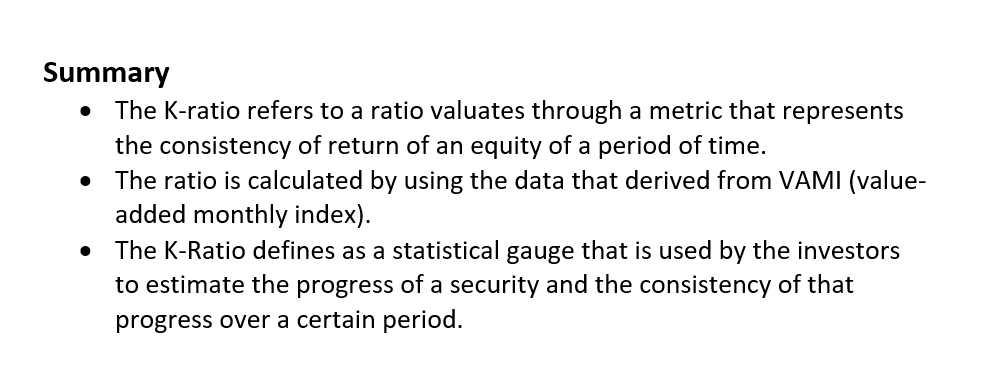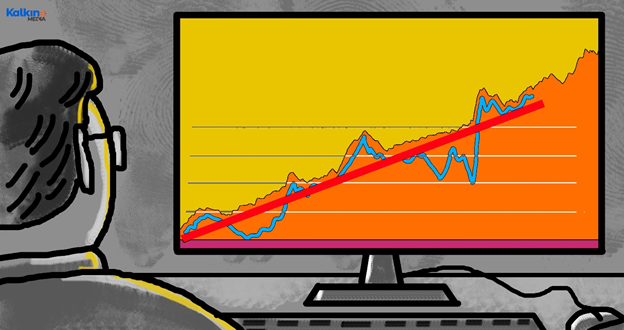# K-Ratio

• Updated on

What is K-ratio?

The K-ratio refers to a ratio valuates through a metric that represents the consistency of return of an equity of a period of time. The ratio is calculated by using the data that derived from VAMI, value-added monthly index is measure that uses linear regression in order to trace the growth of initial US \$1,000 investment in the stock being analyzed.Understanding K-Ratio

The K-Ratio defines as a statistical gauge that is used by the investors to estimate the progress of a security and the consistency of that progress over a certain period. The ratio helps one determine the risk and return of an investment for a particular asset. It also ascertains the consistency of the reward on that risk. The ratio is calculated by using a linear trend for the data of return and measuring the variability of the data. The ratio determines the return vs. risk ratio, taking both return and the risk of an investment.

The ratio is used by the investor because it is not only estimates the return of an investment in a specific security over a particular period but also measure the consistency of that return on an investment over that particular period. If the K-Ratio is high, it shows the better performance of an investment and vice versa. The ratio may be a good tool for an investor or trader for comparing different securities that they may want to estimate through research to know whether it is a good investment or not.History Of K-Ratio

The K-Ratio is introduced by Lars Kestner in 1996 to estimate the overall profitability of a plan or strategy. The ratio was designed and created so that investors or traders can see and study data set in order to get linear line with little digression and this helps investors to look into an investment of an asset with a profitable return. During the past years, some minor adjustments have been made by Kestner to the ratio based on different observed factors including different types of return and return periods.

How To Calculate K-Ratio?

The K-ratio can be calculated through plotting linear regression by using a curve of Value-Added Monthly Index (VAMI) based on arithmetic cumulative return. Here is how it calculated:

• An investor creates a dispersed plot of Value-Added Monthly Index (VAMI) log vs. Number of periods.
• Afterwards, the return is measured through slope based on return’s period including days, weeks, and months. For instance, an investor uses ABC’s monthly stock returns of over 8 years.
• Lastly, an investor measures the risk related with an investment of a specific stock. The risk is measured by standard error. The standard error measures the deviation from the regression line.

The K-ratio is a complimentary ratio represents a slope or standard error which interprets stock return or the associated risk, just like in the Sharpe Ratio. It can be calculated by using the given formula:

K-ratio = (Slope of log Value-Added Monthly Index regression line) / (Standard Regression Error)

Or

K–ratio = (Slope of log Value-Added Monthly Index regression line) / n (Standard Error of the Slope)

Importance Of K-Ratio

Investors and traders are always keen to know the returns of their investments and the consistency of the returns over the specific period. The ratio determines the return vs. risk ratio, taking both return and the risk of an investment. The ratio is calculated by framing a linear regression of the data of returns of an investment over a specific period and measures the slope of standard error that shows the risk associated with an investment, and slope measures the return of an investment over a certain period.

The ratio increases with the rise in slope and decreases with the oversized profit or losses that shows the higher inconsistency. A higher K–ratio indicates a healthier performance of an investment in terms of both better returns and consistency of returns. If the ratio’s value is more than 2.0 is good for investors.

What Can It Tell You?

The ratio is developed by Lars Kestner as a statistical tool in order to analyze returns over a certain period of time. Every investor or trader’s main interest is returns of an investment and its consistency. The ratio is designed by Kestner to estimate the return and the associated risk through analyzing and measuring stability of a portfolio, security’s returns over a specific period. The ratio considered returns of an investment and measure risk through the sequence of those returns. The ratio’s calculation is based on a linear regression and then the outcomes of the linear regression are used in the formula of K-ratio. The slope of a linear regression indicates the return whereas a slope’s standard error shows the risk associated with the returns of an investment.

The ratio measures the consistency of a security’s return over certain period, and the ratio is used to measure and compare the cumulative returns of different stocks over a specific time in order to determine which one is better or worth. The ratio is helpful for analyzing other securities (equities) and strategies instead of single stock.

##### Top ASX Listed Companies

Definition:
We use cookies to ensure that we give you the best experience on our website. If you continue to use this site we will assume that you are happy with it. OK